Courses

# Flow Past a Source - Flow of Ideal Fluids Mechanical Engineering Notes | EduRev

## Mechanical Engineering : Flow Past a Source - Flow of Ideal Fluids Mechanical Engineering Notes | EduRev

The document Flow Past a Source - Flow of Ideal Fluids Mechanical Engineering Notes | EduRev is a part of Mechanical Engineering category.
All you need of Mechanical Engineering at this link: Mechanical Engineering

Flow Past a Source

When a uniform flow is added to that due to a source -

• fluid emitted from the source is swept away in the downstream direction
• stream function and velocity potential for this flow will be the sum of those for uniform flow and source

Stream function;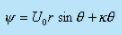Velocity Potential;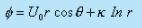so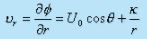and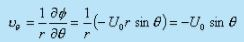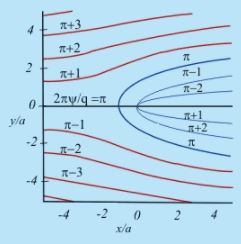Fig 23.1  The streamlines of the flow past a line source for equal increments of 2πψ/q
The Plane coordinates are x/a, y/a where a=k/u

Explanation of Figure

• At the point x = -a, y = 0 fluid velocity is zero.
• This is called stagnation point of the flow
• Here the source flow is turned around by the oncoming uniform flow
• The parabolic streamline passing through stagnation point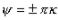seperates uniform flow from the source flow.
• The streamline becomes parallel to x axis as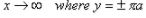Flow Past Vortex

when uniform flow is superimposed with a vortex flow -

• Flow will be asymmetrical about x - axis
• Again stream function and velocity potential will be the sum of those for uniform flow and vortex flow

Stream Function: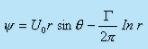Velocity Potential: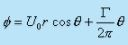so that;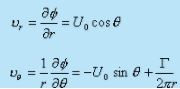Magnus Effect

Flow about a rotating cylinder is equivalent to the combination of flow past a cylinder and a vortex.
As such in addition to superimposed uniform flow and a doublet, a vortex is thrown at the doublet centre which will simulate a rotating cylinder in uniform stream.

The pressure distribution will result in a force, a component of which will culminate in lift force

The phenomenon of generation of lift by a rotating object placed in a stream is known as Magnus effect.

Velocity Potential and Stream Function

The velocity potential and stream functions for the combination of doublet, vortex and uniform flow are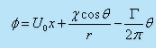(clockwise rotation)              (23.1)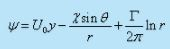(clockwise rotation)              (23.2)

By making use of either the stream function or velocity potential function, the velocity components are (putting x= rcosθ and y= rsinθ )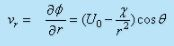(23.3)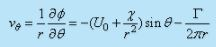(23.4)

Stagnation Points

At the stagnation points the velocity components must vanish. From Eq. (23.3), we get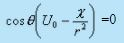(23.5)

Solution :

1. From Eq. (23.5) it is evident that a zero radial velocity component may occur at
•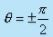and
• along the circle,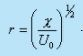Eq. (23.4) depicts that a zero transverse velocity requires(23.6)

At the stagnation point, both radial and transverse velocity components must be zero .

Thus the location of stagnation point occurs at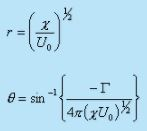There will be two stagnation points since there are two angles for a given sine except for sin-1(±1)

Determination of Stream Line

The streamline passing through these points may be determined by evaluating ψ at these points.

Substitution of the stagnation coordinate (r, θ) into the stream function (Eq. 23.2) yields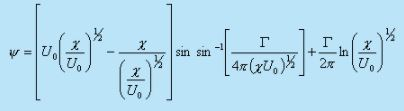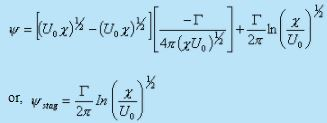(23.7)

Equating the general expression for stream function to the above constant, we get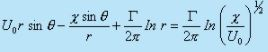By rearranging we can write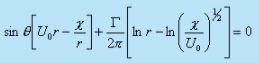(23.8)

All points along the circle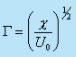satisfy Eq. (23.8) , since for this value of r, each quantity within parentheses in the equation is zero.

Considering the interior of the circle (on which ψ = 0) to be a solid cylinder, the outer streamline pattern is shown in Fig 23.2.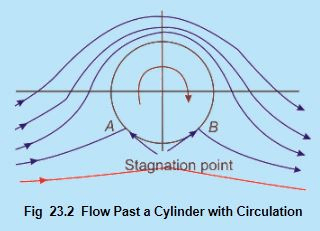At the stagnation point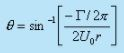The limiting case arises for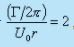, where   θ = sin -1(-1) = -900 and two stagnation points meet at the bottom as shown in Fig. 23.3.

In the case of a circulatory flow past the cylinder, the streamlines are symmetric with respect to the y-axis. The presures at the points on the cylinder surface are symmetrical with respect to the y-axis. There is no symmetry with respect to the x-axis. Therefore a resultant force acts on the cylinder in the direction of the y-axis, and the resultant force in the direction of the x-axis is equal to zero as in the flow without circulation; that is, the D'Alembert paradox takes place here as well. Thus, in the presence of circulation, different flow patterns can take place and, therefore, it is necessary for the uniqueness of the solution, to specify the magnitude of circulation.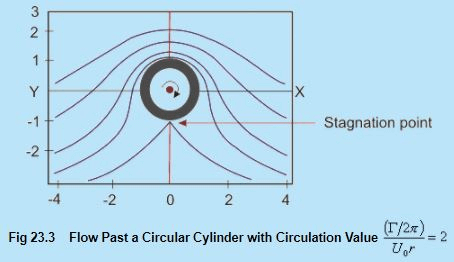However, in all these cases the effects of the vortex and doublet become negligibly small as one moves a large distance from the cylinder.

The flow is assumed to be uniform at infinity.

We have already seen that the change in strength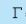of the vortex changes the flow pattern, particularly the position of the stagnation points but the radius of the cylinder remains unchanged.

Lift and Drag for Flow About a Rotating Cylinder

The pressure at large distances from the cylinder is uniform and given by p0.

Deploying Bernoulli's equation between the points at infinity and on the boundary of the cylinder,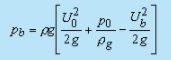(23.9)

Hence,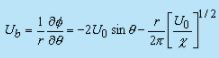(23.10)

From Eqs (23.9) and (23.10) we can write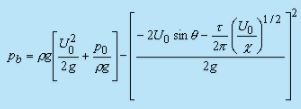(23.11)

The lift may calculated as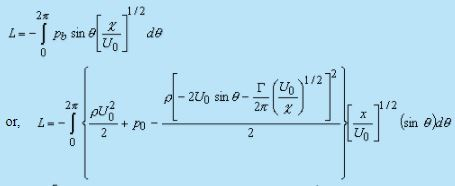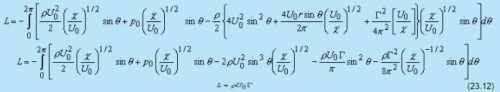The drag force , which includes the multiplication by cosθ (and integration over 2π) is zero.

• Thus the inviscid flow also demonstrates lift.

•  lift becomes a simple formula involving only the density of the medium, free stream velocity and circulation.

•  in two dimensional incompressible steady flow about a boundary of any shape, the lift is always a product of these three quantities.----- Kutta- Joukowski theorem

Aerofoil Theory

Aerofoils are streamline shaped wings which are used in airplanes and turbo machinery. These shapes are such that the drag force is a very small fraction of the lift. The following nomenclatures are used for defining an aerofoil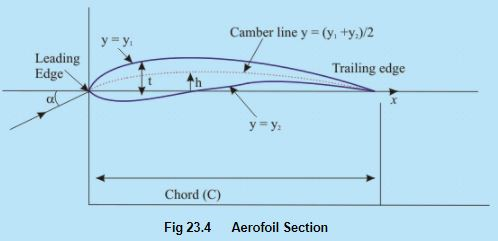• The chord (C) is the distance between the leading edge and trailing edge.
• The length of an aerofoil, normal to the cross-section (i.e., normal to the plane of a paper) is called the span of a aerofoil.
• The camber line represents the mean profile of the aerofoil. Some important geometrical parameters for an aerofoil are the ratio of maximum thickness to chord (t/C) and the ratio of maximum camber to chord (h/C). When these ratios are small, an aerofoil can be considered to be thin. For the analysis of flow, a thin aerofoil is represented by its camber.

The theory of thick cambered aerofoils uses a complex-variable mapping which transforms the inviscid flow across a rotating cylinder into the flow about an aerofoil shape with circulation.

Flow Around a Thin Aerofoil

• Thin aerofoil theory is based upon the superposition of uniform flow at infinity and a continuous distribution of clockwise free vortex on the camber line having circulation density γ (s) per unit length .
• The circulation density  γ (s)  should be such that the resultant flow is tangent to the camber line at every point.
• Since the slope of the camber line is assumed to be small,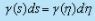. The total circulation around the profile is given by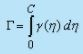(23.13)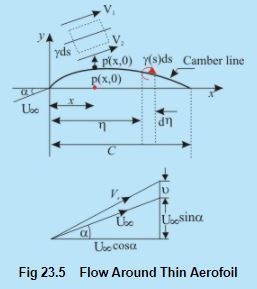A vortical motion of strength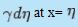develops a velocity at the point p which may be expressed as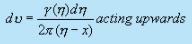The total induced velocity in the upward direction at point p due to the entire vortex distribution along the camber line is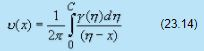For a small camber (having small α), this expression is identically valid for the induced velocity at point p' due to the vortex sheet of variable strength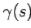on the camber line. The resultant velocity due to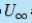and v(x) must be tangential to the camber line so that the slope of a camber line may be expressed as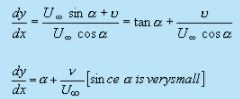(23.15)

From Eqs (23.14) and (23.15) we can write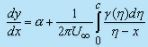Consider an element ds on the camber line. Consider a small rectangle (drawn with dotted line) around ds. The upper and lower sides of the rectangle are very close to each other and these are parallel to the camber line. The other two sides are normal to the camber line. The circulation along the rectangle is measured in clockwise direction as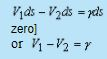[normal component of velocity at the camber line should be

[normal component of velocity at the camber line should be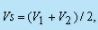it can be rewritten as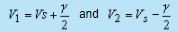f v is very small   [v << U.], Vbecomes equal to U. The difference in velocity across the camber line brought about by the vortex sheet of variable strength y (s) causes pressure difference and generates lift force.

Generation of Vortices Around a Wing

• The lift around an aerofoil is generated following Kutta-Joukowski theorem . Lift is a product of ρ , U. and the circulation.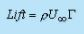• When the motion of a wing starts from rest, vortices are formed at the trailing edge.
• At the start, there is a velocity discontinuity at the trailing edge. This is eventual because near the trailing edge, the velocity at the bottom surface is higher than that at the top surface. This discrepancy in velocity culminates in the formation of vortices at the trailing edge.
• Figure 23.6(a) depicts the formation of starting vortex by impulsively moving aerofoil. However, the starting vortices induce a counter circulation as shown in Figure 23.6(b). The circulation around a path (ABCD) enclosing the wing and just shed (starting) vortex must be zero. Here we refer to Kelvin's theorem once again.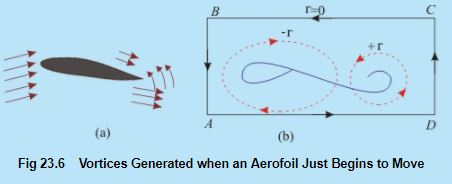• Initially, the flow starts with the zero circulation around the closed path. Thereafter, due to the change in angle of attack or flow velocity, if a fresh starting vortex is shed, the circulation around the wing will adjust itself so that a net zero vorticity is set around the closed path.
• Real wings have finite span or finite aspect ratio (AR) λ , defined as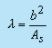(23.16 )

where b is the span length, As is the plan form area as seen from the top..

• For a wing of finite span, the end conditions affect both the lift and the drag. In the leading edge region, pressure at the bottom surface of a wing is higher than that at the top surface. The longitudinal vortices are generated at the edges of finite wing owing to pressure differences between the bottom surface directly facing the flow and the top surface.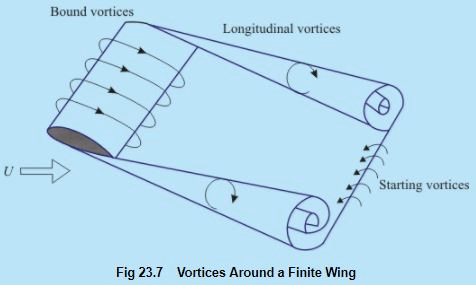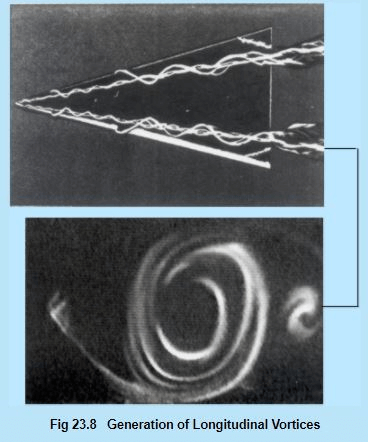Offer running on EduRev: Apply code STAYHOME200 to get INR 200 off on our premium plan EduRev Infinity!

,

,

,

,

,

,

,

,

,

,

,

,

,

,

,

,

,

,

,

,

,

;